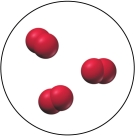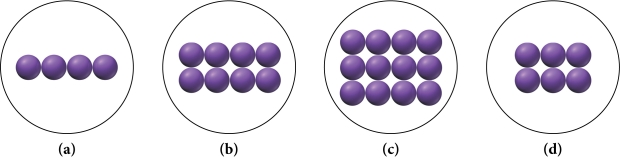# Problem: Under certain conditions sodium can react with oxygen to form sodium oxide according to the reaction: 4 Na(s) + O2(g) → 2 Na2O(s)A flask contains the amount of oxygen represented by the diagram below.Which image below best represents the amount of sodium required to completely react with all of the oxygen in the flask according to the equation?

###### FREE Expert Solution

We are asked to identify the image below best represents the amount of sodium required to completely react with all of the oxygen in the flask according to the equation.

4 Na(s) + O2(g) → 2 Na2O(s)

Given:

3 molecule O2

93% (15 ratings)###### Problem Details

Under certain conditions sodium can react with oxygen to form sodium oxide according to the reaction: 4 Na(s) + O2(g) → 2 Na2O(s)

A flask contains the amount of oxygen represented by the diagram below.Which image below best represents the amount of sodium required to completely react with all of the oxygen in the flask according to the equation?Frequently Asked Questions

What scientific concept do you need to know in order to solve this problem?

Our tutors have indicated that to solve this problem you will need to apply the Stoichiometry concept. You can view video lessons to learn Stoichiometry. Or if you need more Stoichiometry practice, you can also practice Stoichiometry practice problems.

What professor is this problem relevant for?

Based on our data, we think this problem is relevant for Professor Blankenship's class at UGA.